# 商品亲和性分析

• 支持度：支持度指的是数据集中规则应验的次数。（商品交易中同时买A商品和B商品的交易数量【支持度也可以为次数/交易总量】）
• 置信度：置信度代表的是规则的准确性如何。（既买A商品又买B商品的数量除以买A商品的数量）

``````import pandas as pd
from itertools import combinations

features = ["bread", "milk", "cheese", "apple", "banana"]
``````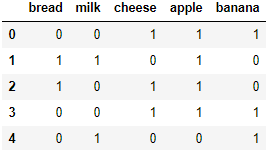``````import numpy as np

X = np.c_[np.random.random(100) < 0.3, np.random.random(100) < 0.5,
np.random.random(100) < 0.2, np.random.random(100) < 0.25,
np.random.random(100) < 0.5]
X[X.sum(axis=1) == 0, 4] = 1
print(X[:5])
np.savetxt("affinity_dataset.txt", X, fmt="%d")
``````

``````data = []

for i, j in combinations(range(df.shape), 2):
support = df.iloc[:, [i, j]].all(axis=1).sum()
data.append((i, j, support))
data.append((j, i, support))
data = pd.DataFrame(data, columns=["A", "B", "support"])
data.A = pd.Categorical.from_codes(data.A, features)
data.B = pd.Categorical.from_codes(data.B, features)
``````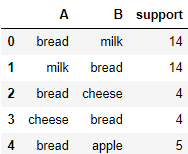``````data['support2'] = data.support/df.shape
``````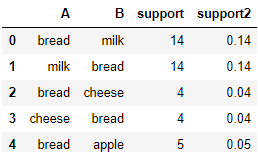``````num_occurances = df.sum()
num_occurances
``````
``````bread     27
milk      46
cheese    41
apple     36
banana    59
dtype: int64
``````

``````data["confidence"] = data.support/num_occurances[data.A].values
``````
ABsupportsupport2confidence

``````data.sort_values(["support", "confidence"], ascending=False, inplace=True)
``````
ABsupportsupport2confidence
16cheesebanana270.270.658537
17bananacheese270.270.457627
15applecheese250.250.694444
14cheeseapple250.250.609756
18applebanana210.210.583333

# 基于关联规则的Apriori算法

Agrawal等人在1993年提出关联规则的概念后，又在1994年提出了基于关联规则的 Apriori 算法，至今 Apriori 仍是关联规则挖掘的重要算法。

Apriori算法的三个基本指标是：

• 支持度：1个或多个商品组合出现的次数，或与总次数之间的比例。
• 置信度: 购买A商品的订单中，同时购买B商品的订单比例。
• 提升度：提升度 (A→B)= 置信度 (A→B)/ 支持度 (B)

## 关联规则中的支持度、置信度和提升度

``````data = [('牛奶', '面包', '尿布'),
('可乐', '面包', '尿布', '啤酒'),
('牛奶', '尿布', '啤酒', '鸡蛋'),
('面包', '牛奶', '尿布', '啤酒'),
('面包', '牛奶', '尿布', '可乐')]
``````

``````import pandas as pd
import numpy as np
from itertools import chain,combinations

columns = pd.Index(chain(*data)).unique()
zero_matrix = np.zeros((len(data), len(columns)), dtype='int8')
for i, rows in enumerate(data):
t = columns.get_indexer(rows)
zero_matrix[i, t] = 1
df = pd.DataFrame(zero_matrix, columns=columns)
df
``````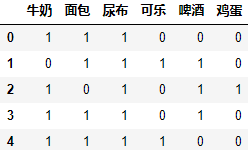### 支持度

``````rule = []
for k in range(1, len(columns)+1):
for keys in combinations(columns, k):
value = df.loc[:, keys].all(axis=1).sum()
if value > 0:
rule.append((k, keys, value))
rule = pd.DataFrame(rule, columns=["k", "rule", "support"])
rule['support2'] = rule.support/df.shape
rule.sample(7)
``````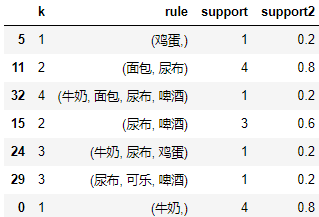``````support = rule.set_index("rule").support2
``````

### 置信度

``````support[('牛奶', '啤酒')]/support[('牛奶',)]
``````
``````0.5
``````

``````support[('牛奶', '啤酒')]/support[('啤酒',)]
``````
``````0.6666666666666667
``````

### 提升度

1. 提升度 (A→B)>1：代表有提升；
2. 提升度 (A→B)=1：代表有没有提升，也没有下降；
3. 提升度 (A→B)<1：代表有下降。

``````support[('牛奶', '啤酒')]/support[('牛奶',)]/support[('啤酒',)]
``````
``````0.8333333333333334
``````

``````support[('尿布', '可乐')]/support[('可乐',)]/support[('尿布',)]
``````
``````1.0
``````

## Apriori 算法的简介与工具使用

Apriori 算法就是查找频繁项集 (frequent itemset) 的过程。

Apriori 算法的递归流程：

1. K=1，计算 K 项集的支持度；
2. 筛选掉小于最小支持度的项集；
3. 如果项集为空，则对应 K-1 项集的结果为最终结果。
如果项集不为空，K=K+1，回到第一步重复执行。

``````itemset = rule.query("support2>=0.5")
itemset
``````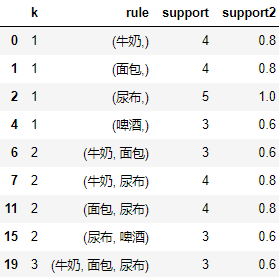efficient-apriori工具包可以很方便的使用Apriori 算法，使用如下命令安装：

pip install efficient-apriori

``````from efficient_apriori.itemsets import itemsets_from_transactions

itemsets, num_trans = itemsets_from_transactions(data, min_support=0.5)
print("总条数：",num_trans)
itemsets
``````
``````总条数： 5
{1: {('牛奶',): 4, ('面包',): 4, ('尿布',): 5, ('啤酒',): 3},
2: {('尿布', '牛奶'): 4, ('尿布', '面包'): 4, ('牛奶', '面包'): 3, ('啤酒', '尿布'): 3},
3: {('尿布', '牛奶', '面包'): 3}}
``````

``````from efficient_apriori.rules import generate_rules_apriori

rules = list(generate_rules_apriori(
itemsets, min_confidence=0.5, num_transactions=num_trans))
rules
``````
``````[{牛奶} -> {尿布},
{尿布} -> {牛奶},
{面包} -> {尿布},
{尿布} -> {面包},
{面包} -> {牛奶},
{牛奶} -> {面包},
{尿布} -> {啤酒},
{啤酒} -> {尿布},
{牛奶, 面包} -> {尿布},
{尿布, 面包} -> {牛奶},
{尿布, 牛奶} -> {面包},
{面包} -> {尿布, 牛奶},
{牛奶} -> {尿布, 面包},
{尿布} -> {牛奶, 面包}]
``````

``````from efficient_apriori import apriori

itemsets, rules = apriori(data, min_support=0.5,  min_confidence=0.5)
print(itemsets)
print("-----")
print(rules)
``````
``````{1: {('牛奶',): 4, ('面包',): 4, ('尿布',): 5, ('啤酒',): 3}, 2: {('尿布', '牛奶'): 4, ('尿布', '面包'): 4, ('牛奶', '面包'): 3, ('啤酒', '尿布'): 3}, 3: {('尿布', '牛奶', '面包'): 3}}
-----
[{牛奶} -> {尿布}, {尿布} -> {牛奶}, {面包} -> {尿布}, {尿布} -> {面包}, {面包} -> {牛奶}, {牛奶} -> {面包}, {尿布} -> {啤酒}, {啤酒} -> {尿布}, {牛奶, 面包} -> {尿布}, {尿布, 面包} -> {牛奶}, {尿布, 牛奶} -> {面包}, {面包} -> {尿布, 牛奶}, {牛奶} -> {尿布, 面包}, {尿布} -> {牛奶, 面包}]
``````

``````features = ["bread", "milk", "cheese", "apple", "banana"]
``````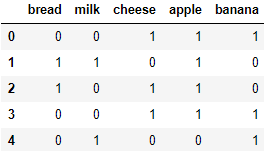``````mask = df.columns
data = [tuple(mask[rows == 1]) for rows in df.values]
data[:5]
``````
``````[('cheese', 'apple', 'banana'),
('cheese', 'apple', 'banana'),
('milk', 'banana')]
``````

``````itemsets, rules = apriori(data, min_support=0.2,  min_confidence=0.1)
print(itemsets)
print("-----")
print(rules)
``````
``````{1: {('banana',): 59, ('apple',): 36, ('cheese',): 41, ('milk',): 46, ('bread',): 27}, 2: {('apple', 'banana'): 21, ('apple', 'cheese'): 25, ('banana', 'cheese'): 27}}
-----
[{banana} -> {apple}, {apple} -> {banana}, {cheese} -> {apple}, {apple} -> {cheese}, {cheese} -> {banana}, {banana} -> {cheese}]
``````

# FP-Growth算法

## 简介与工具包使用

FP-Growth算法是基于Apriori改进的关联挖掘的算法，Apriori 在计算的过程有哪些缺点呢？

1. 采用排列组合的方式，可能产生大量的候选集。
2. 每次计算每个项集的支持度都需要重新扫描数据集。

FP-Growth算法的特点是：

1. 创建了一棵 FP 树来存储频繁项集，在创建前对不满足最小支持度的项进行删除。
2. 整个生成过程只遍历数据集 2 次。

FP-Growth算法的原理较为复杂，下面我们先直接使用现有的包pyfpgrowth，直接使用FP-GROWTH算法进行关联规则挖掘。

pyfpgrowth计算最小支持度是20%的频繁集项(pyfpgrowth的支持度用的是数量)：

``````import pyfpgrowth as fp

patterns = fp.find_frequent_patterns(data, int(0.2*df.shape))
patterns
``````
``````{('bread',): 27,
('apple', 'banana'): 21,
('apple', 'cheese'): 25,
('cheese',): 41,
('banana', 'cheese'): 27,
('milk',): 46,
('banana',): 59}
``````

pyfpgrowth计算最小支持度是10%的频繁规则：

``````fp.generate_association_rules(patterns, 0.1)
``````
``````{('banana',): (('cheese',), 0.4576271186440678),
('cheese',): (('banana',), 0.6585365853658537)}
``````

``````data = [('牛奶', '面包', '尿布'),
('可乐', '面包', '尿布', '啤酒'),
('牛奶', '尿布', '啤酒', '鸡蛋'),
('面包', '牛奶', '尿布', '啤酒'),
('面包', '牛奶', '尿布', '可乐')]
patterns = fp.find_frequent_patterns(data, int(0.5*len(data)))
patterns
``````
``````{('可乐',): 2,
('可乐', '面包'): 2,
('可乐', '尿布'): 2,
('可乐', '尿布', '面包'): 2,
('啤酒', '面包'): 2,
('啤酒', '尿布', '面包'): 2,
('啤酒', '牛奶'): 2,
('啤酒', '尿布', '牛奶'): 2,
('啤酒', '尿布'): 3,
('牛奶',): 4,
('尿布', '牛奶'): 4,
('牛奶', '面包'): 2,
('尿布', '牛奶', '面包'): 2,
('面包',): 4,
('尿布', '面包'): 4,
('尿布',): 5}
``````
``````fp.generate_association_rules(patterns, 0.5)
``````
``````{('可乐',): (('尿布', '面包'), 1.0),
('面包',): (('尿布',), 1.0),
('可乐', '尿布'): (('面包',), 1.0),
('可乐', '面包'): (('尿布',), 1.0),
('尿布', '面包'): (('牛奶',), 0.5),
('啤酒', '尿布'): (('牛奶',), 0.6666666666666666),
('啤酒', '面包'): (('尿布',), 1.0),
('牛奶',): (('尿布', '面包'), 0.5),
('啤酒', '牛奶'): (('尿布',), 1.0),
('尿布', '牛奶'): (('面包',), 0.5),
('尿布',): (('面包',), 0.8),
('牛奶', '面包'): (('尿布',), 1.0)}
``````

## FP-Growth算法的原理

1. 创建项头表，对于满足最小支持度的单个项(K=1项集)按照支持度从高到低进行排序，同时删除不满足最小支持度的项。
2. 构造 FP 树，根节点记为NULL节点，再次扫描数据集，对于每一条数据，按照支持度从高到低的顺序进行创建节点（项头表中的排序结果），并更新节点计数和项头表的链表。
3. 通过 FP 树挖掘频繁项集，具体的操作运用“条件模式基”

### FP Tree数据结构

FP-Growth 算法涉及的数据结构包括三部分：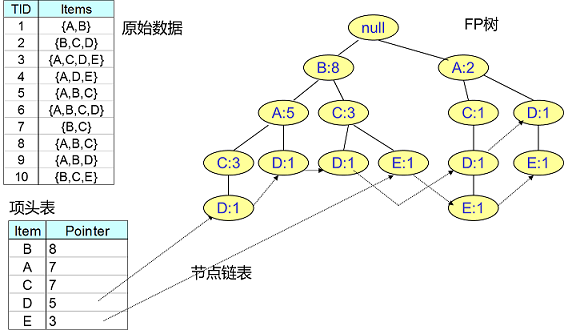1. 项头表：

里面记录了所有的1项频繁集出现的次数，按照次数降序排列。比如上图中B在所有10组数据中出现了8次，因此排在第一位。

2. FP Tree：

它将原始数据集映射到了内存中的一颗FP树

3. 节点链表：

所有项头表里的1项频繁集都是一个节点链表的头，它依次指向FP树中该1项频繁集出现的位置。这样做主要是方便项头表和FP Tree之间的联系查找和更新。

### 项头表的建立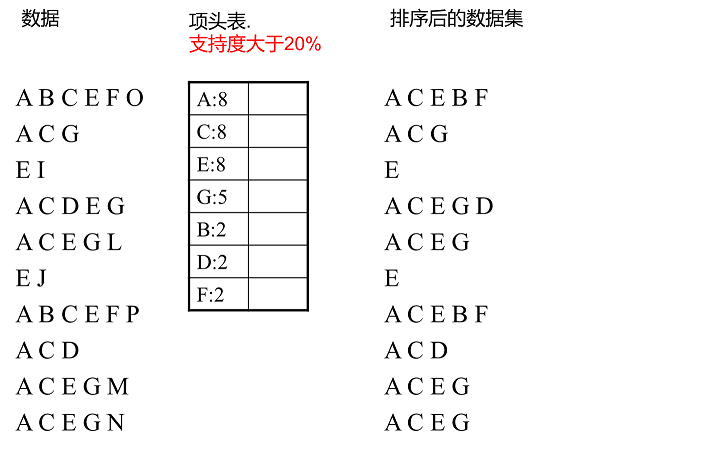### FP Tree的建立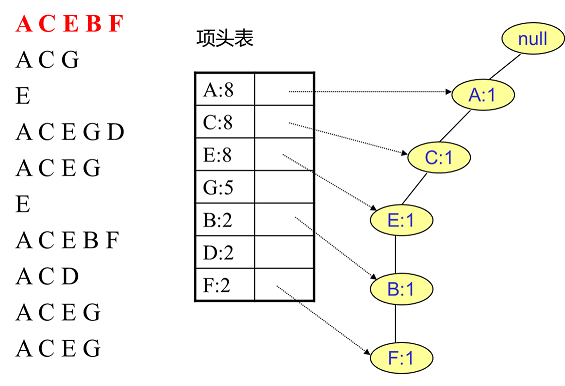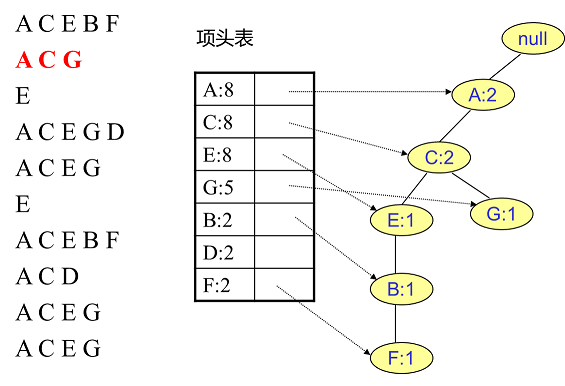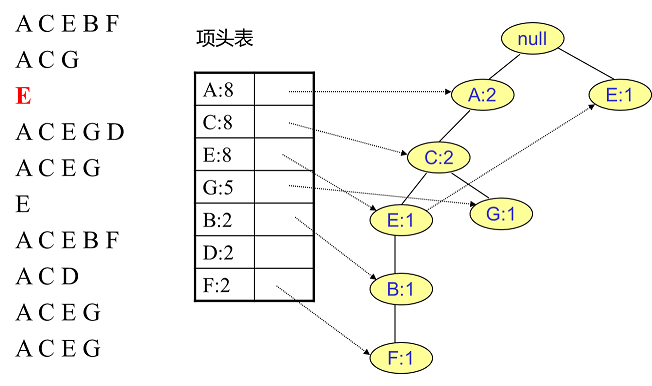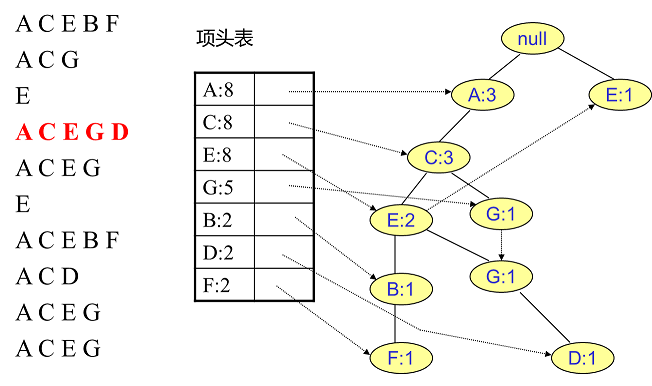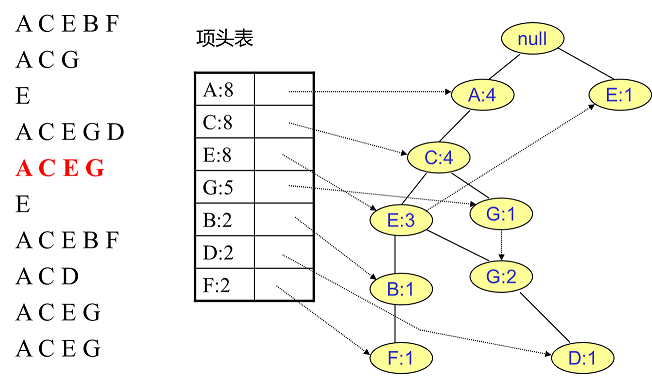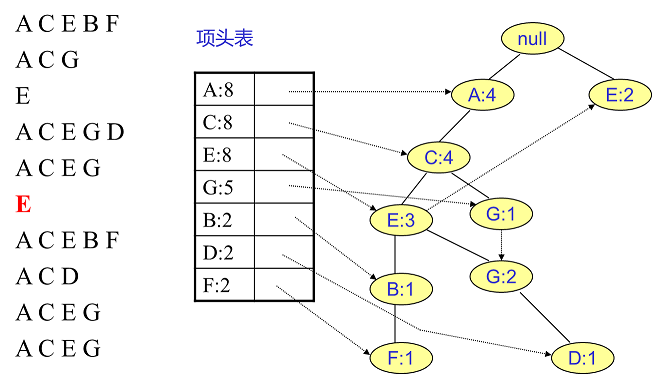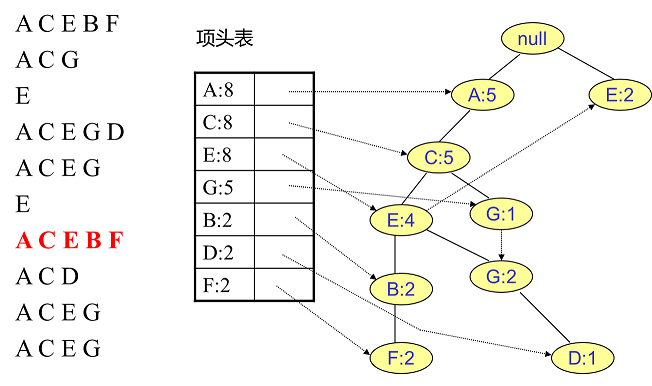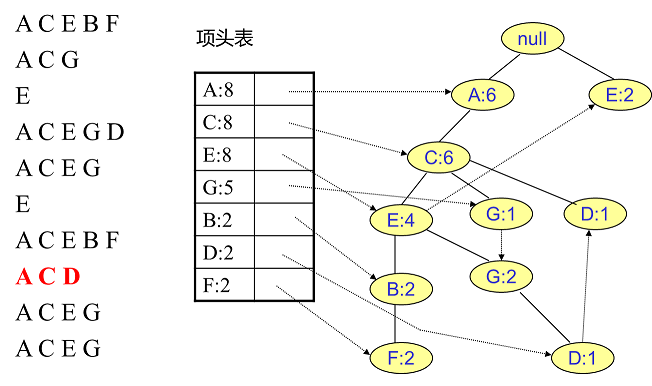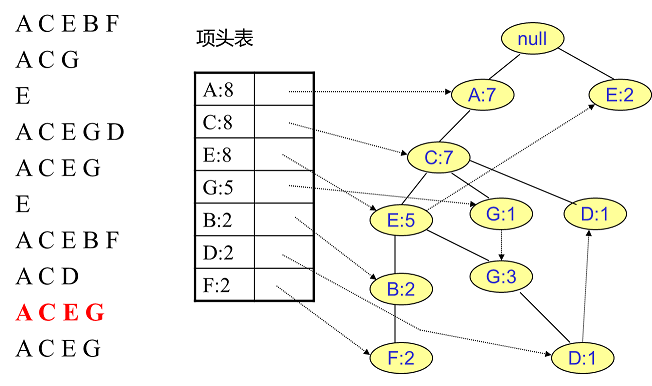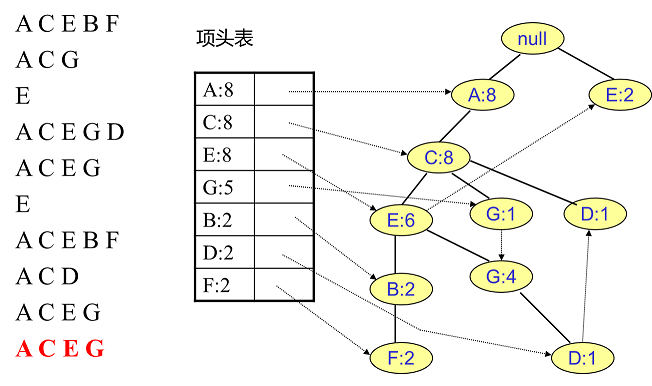### FP Tree的挖掘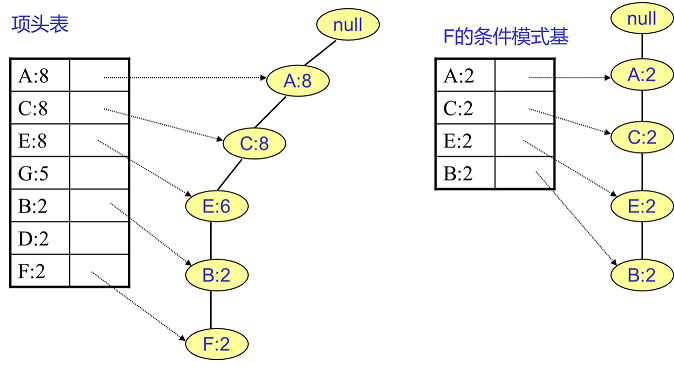D节点有两个叶子节点比F节点复杂一些。首先得到的FP子树如下图左，接着将所有的祖先节点计数设置为叶子节点的计数，即变成{A:2, C:2,E:1 G:1,D:1, D:1}，此时E节点和G节点由于在条件模式基里面的支持度低于阈值被删除掉，最终在去除低支持度节点并不包括叶子节点后D的条件模式基为{A:2, C:2}。通过它，可得到F的频繁2项集为{A:2,D:2}, {C:2,D:2}。递归合并二项集，得到频繁三项集为{A:2,C:2,D:2}。D对应的最大的频繁项集为频繁3项集。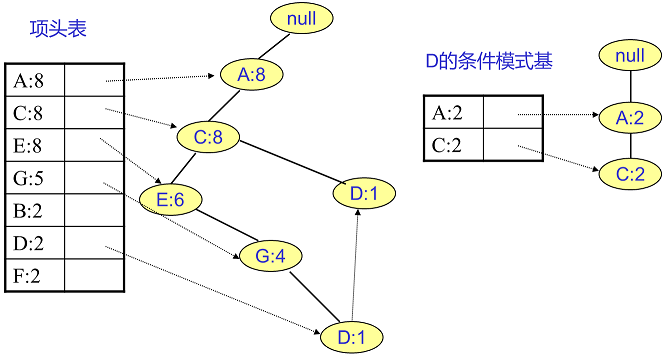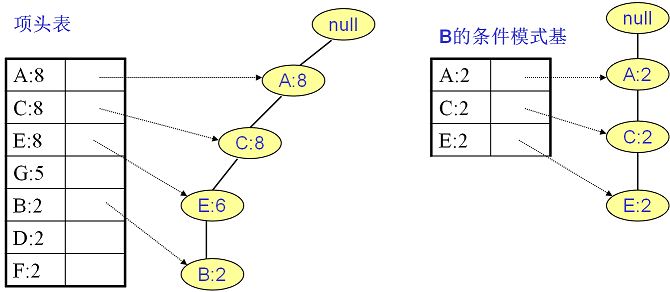G的条件模式基如下图右边，递归挖掘到G的最大频繁项集为频繁4项集{A:5, C:5, E:4,G:4}：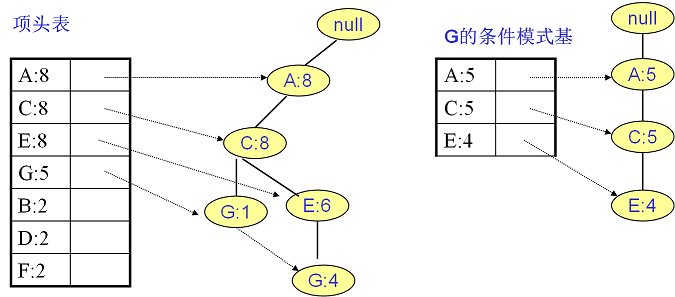E的条件模式基如下图右边，递归挖掘到E的最大频繁项集为频繁3项集{A:6, C:6, E:6}。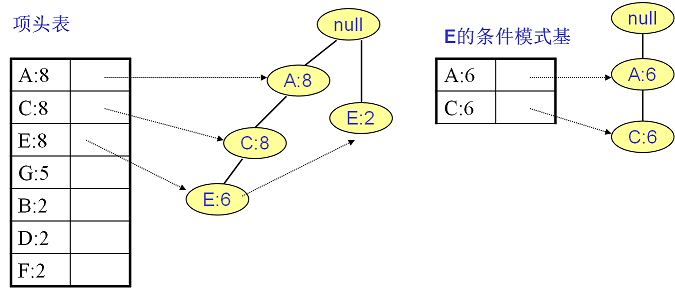C的条件模式基如下图右边，递归挖掘到C的最大频繁项集为频繁2项集{A:8, C:8}。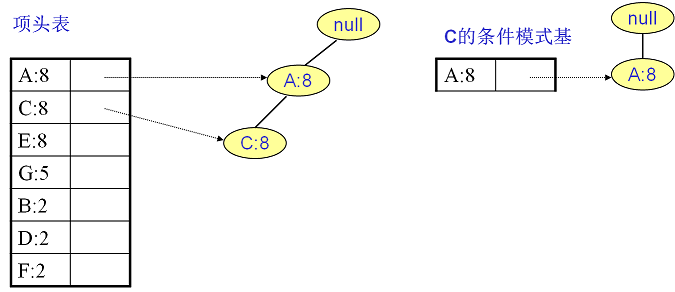A的条件模式基为空，因此不用去挖掘。

# 案例：挖掘导演是如何选择演员的

## 豆瓣爬虫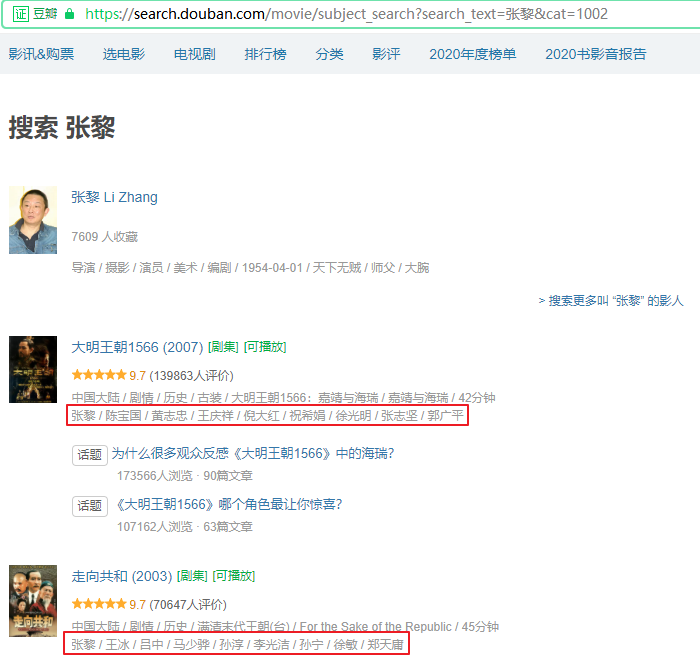selenium需要pip安装，我当前电脑未安装谷歌游览器，所以使用了PhantomJS。

Selenium和相应驱动的安装方法详见：https://blog.csdn.net/as604049322/article/details/114157526

``````from selenium import webdriver
from urllib.parse import quote
import time
from lxml import etree
import pandas as pd
from efficient_apriori import apriori

director = '张黎'
start = 0
url_template = 'https://search.douban.com/movie/subject_search?search_text={}&cat=1002&start={}'
driver = webdriver.PhantomJS()
``````
``````result = []
while start < 1000:
url = url_template.format(quote(director), start)
print(url)
start += 15
driver.get(url)
time.sleep(1)
html = driver.find_element_by_xpath("//*").get_attribute("outerHTML")
html = etree.HTML(html)
movie_list = html.xpath("//div[@class='item-root']//div[@class='detail']")
for movie in movie_list:
name = movie.xpath(".//a[@class='title-text']/text()")
actors = movie.xpath("./div[@class='meta abstract_2']/text()")
# 不是目标导演导演的电影则跳过
if len(actors) == 0 or not actors.startswith(director):
continue
result.append((name.replace("\u200e ", ""),
actors.replace(" ", "")[len(director)+1:]))
if len(movie_list) < 14:
break
df = pd.DataFrame(result, columns=["name", "actors"])
# 清空演员为空的
df = df.query("actors!=''")
df
``````
``````https://search.douban.com/movie/subject_search?search_text=%E5%BC%A0%E9%BB%8E&cat=1002&start=0
https://search.douban.com/movie/subject_search?search_text=%E5%BC%A0%E9%BB%8E&cat=1002&start=15
https://search.douban.com/movie/subject_search?search_text=%E5%BC%A0%E9%BB%8E&cat=1002&start=30
``````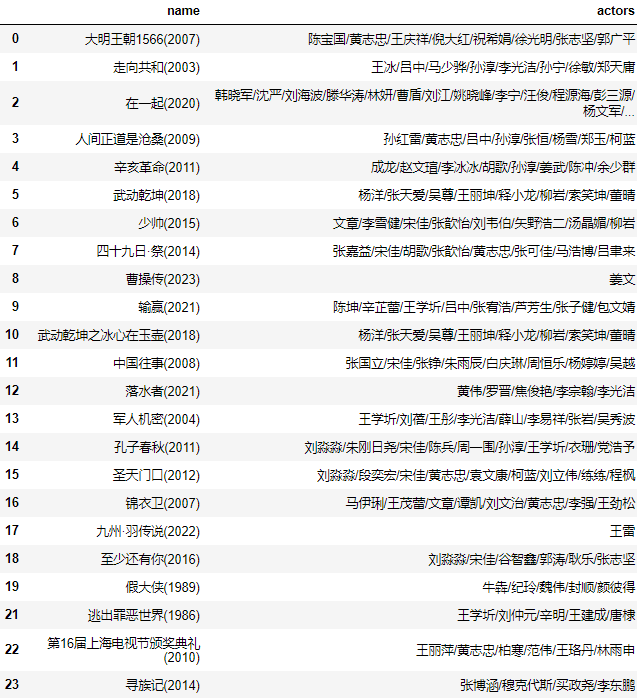``````data = df.actors.str.split("/").to_list()
print(data)
``````
``````[['陈宝国', '黄志忠', '王庆祥', '倪大红', '祝希娟', '徐光明', '张志坚', '郭广平'], ['王冰', '吕中', '马少骅', '孙淳', '李光洁', '孙宁', '徐敏', '郑天庸'], ['韩晓军', '沈严', '刘海波', '滕华涛', '林妍', '曹盾', '刘江', '姚晓峰', '李宁', '汪俊', '程源海', '彭三源', '杨文军', '杨阳', '张嘉益', '周一围', '谭卓', '张天爱', '何蓝逗', '梅婷', '雷佳音', '倪妮'], ['孙红雷', '黄志忠', '吕中', '孙淳', '张恒', '杨雪', '郑玉', '柯蓝'], ['成龙', '赵文瑄', '李冰冰', '胡歌', '孙淳', '姜武', '陈冲', '余少群'], ['杨洋', '张天爱', '吴尊', '王丽坤', '释小龙', '柳岩', '索笑坤', '董晴'], ['文章', '李雪健', '宋佳', '张歆怡', '刘韦伯', '矢野浩二', '汤晶媚', '柳岩'], ['张嘉益', '宋佳', '胡歌', '张歆怡', '黄志忠', '张可佳', '马浩博', '吕聿来'], ['姜文'], ['陈坤', '辛芷蕾', '王学圻', '吕中', '张宥浩', '芦芳生', '张子健', '包文婧'], ['杨洋', '张天爱', '吴尊', '王丽坤', '释小龙', '柳岩', '索笑坤', '董晴'], ['张国立', '宋佳', '张铮', '朱雨辰', '白庆琳', '周恒乐', '杨婷婷', '吴越'], ['黄伟', '罗晋', '焦俊艳', '李宗翰', '李光洁'], ['王学圻', '刘蓓', '王彤', '李光洁', '薛山', '李易祥', '张岩', '吴秀波'], ['刘淼淼', '朱刚日尧', '宋佳', '陈兵', '周一围', '孙淳', '王学圻', '衣珊', '党浩予'], ['刘淼淼', '段奕宏', '宋佳', '黄志忠', '袁文康', '柯蓝', '刘立伟', '练练', '程枫'], ['马伊琍', '王茂蕾', '文章', '谭凯', '刘文治', '黄志忠', '李强', '王劲松'], ['王雷'], ['刘淼淼', '宋佳', '谷智鑫', '郭涛', '耿乐', '张志坚'], ['牛犇', '纪玲', '魏伟', '封顺', '颜彼得'], ['王学圻', '刘仲元', '辛明', '王建成', '唐棣'], ['王丽萍', '黄志忠', '柏寒', '范伟', '王珞丹', '林雨申'], ['张博涵', '穆克代斯', '买政尧', '李东鹏']]
``````

## 关联规则挖掘

``````# 挖掘频繁项集和关联规则
itemsets = fp.find_frequent_patterns(data, 3)
rules = fp.generate_association_rules(itemsets, 1)
print(itemsets)
print("--------")
print(rules)
``````
``````{('吕中',): 3, ('李光洁',): 3, ('张天爱',): 3, ('柳岩',): 3, ('刘淼淼',): 3, ('刘淼淼', '宋佳'): 3, ('孙淳',): 4, ('王学圻',): 4, ('黄志忠',): 6, ('宋佳',): 6}
--------
{('刘淼淼',): (('宋佳',), 1.0)}
``````